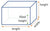# How many gallons of water is in my pool?

enero 20, 2023Determining the exact number of gallons of water in your pool can be a bit tricky, as it depends on a number of factors such as the shape and size of your pool, as well as the water level. However, there are a few basic methods you can use to estimate the number of gallons of water in your pool.
The first method is to use a pool volume calculator. These calculators are available online and can help you estimate the number of gallons of water in your pool based on the shape, size, and depth of your pool. Simply enter the dimensions of your pool and the calculator will give you an estimate of the number of gallons of water it holds.
Another method is to use the pool's dimensions and depth to calculate the number of gallons. This can be done by multiplying the length, width, and average depth of the pool together, then multiplying that number by 7.5 (which is the number of gallons in one cubic foot of water). For example, if your pool is 20 feet long, 10 feet wide, and has an average depth of 5 feet, the calculation would be: (20 x 10 x 5) x 7.5 = 7,500 gallons.
A third way is to use a pool water meter, a device that can measure the amount of water in a pool. This device is inserted into the pool water and will give you the exact amount of water in gallons.
It is important to note that these methods are just estimates and the actual number of gallons of water in your pool may vary slightly. Additionally, it is important to keep in mind that the water level in your pool can change over time due to factors such as evaporation and water loss. Therefore, it's a good idea to periodically check the water level in your pool and add water as needed.
In conclusion, determining the exact number of gallons of water in your pool can be a bit tricky, but there are a few basic methods you can use to estimate it. You can use a pool volume calculator, use the pool's dimensions and depth to calculate the number of gallons, or use a pool water meter. Keep in mind that the actual number of gallons of water in your pool may vary slightly and it's a good idea to periodically check the water level in your pool and add water as needed.

#### Dejar un comentario

Los comentarios se aprobarán antes de mostrarse.